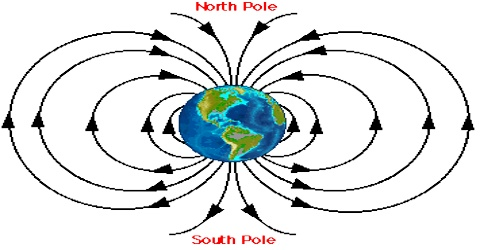# Gravitational Field Explanation

Gravitational field

Gravitational force of attraction between two bodies acts without any physical contact between these bodies. From the idea of gravitational field nature of this force can be understood well.

According to this idea, the region around a body acquires a special property due to the presence of that body. Because of this property if a body is brought near to it then gravitational force acts on it. In whatever region if this property exists, then it is understood that there is gravitational field in that region. So, gravitational field act as the media for transferring of gravitational force.

Definition: The space around an object in which its gravitational attraction is felt is called the gravitational field.

It is the gravitational field due to which a body exerts force on any other body placed inside the field. It is measured in units of force per mass, usually newtons per kilogram (N/kg). A gravitational field is a type of force field and is analogous to electric and magnetic fields for electrically charged particles and magnets, respectively.In figure, there is a body of large mass at point A inside a space bounded by the curved surface. Because of it gravitational field has been created inside the space. Now, if body of any mass is kept at point B or C, inside the surface gravitational force will act on it. The more the distance of B and C is increases from A, magnitude of the force decreases accordingly. Actually gravitational field extends upto infinity.

Influence of gravitational field of a body does not remain same everywhere. Its influence is different at different points. Its effect or influence is measured by gravitational field intensity or strength. By placing a unit mass at a point in gravitational field, gravitational field is measured by the force acting on that unit mass.